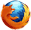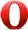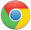# JavaScript abs() 方法JavaScript Math 对象

Math.abs(-7.25);

7.25

## 定义和用法

abs() 方法可返回一个数的绝对值。

## 浏览器支持Math.abs(x)

x 必需。必须是一个数值。

## 返回值

Number x 的绝对值。如果 x 不是数字返回 NaN,如果 x 为 null 返回 0。

## 技术细节

 JavaScript 版本： 1

## 实例

var a=Math.abs(7.25);
var b=Math.abs(-7.25);
var c=Math.abs(null);
var d=Math.abs("Hello");
var e=Math.abs(2+3);

7.25
7.25
0
NaN
5JavaScript Math 对象